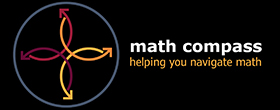# Math Help## Math Support

Appointment-based and on-demand tutoring for foundational math courses and concepts.

### Math Drop-In

• Do you need help with a math question?
• Do you want homework support?
• Are you looking for a quick math consult?
• Are you struggling with basic math?

### Math Tutoring Appointments

• Are you taking a math course?
• Are you struggling with math in a course?

### PASS Sessions

• Are you taking first-semester business math (MATH 10083 / 10084) or MATH  MA179?
• Do you want a group session to prepare for tests and exams?

### Fennell Campus

In-Person Support at the Learning Support Centre (Room C122)

or via Zoom

Monday to Friday: 9am - 4pm
*Starts Monday, September 18### Stoney Creek Campus

In-person support in the Library or via Zoom

Monday, Wednesday, and Friday: 9am – 5pm

Tuesday and Thursday: 10am – 6pm

* Starts Monday, September 11

## Math Resources

### Math Minutes VideosThe Math Minutes videos are a series of videos that cover some of the basics of Mathematics, including:

• Basic Operations: Multiplication without a calculator, long division, order of operations, simplifying radicals without a calculator
• Fractions: Addition, subtraction, multiplication, and division of fractions and mixed numbers
• Decimals and Percent: Decimals and rounding, percentages
• Algebra: Basic algebra, exponent rules, factoring
• Linear Equations: graphing a line using a table of values and using slope and y-intercept
• Trigonometry: Pythagorean Theorem, Trigonometric ratios
• Logarithms: Definition, laws, simplifying logarithms, expanded forms

### Math Compass VideosCheck out the Math Compass videos for helpful strategies and how to study for and take an online math test.

Watch: How to study for an online math test

Watch: How to take an online math test

Watch: Math learning strategies